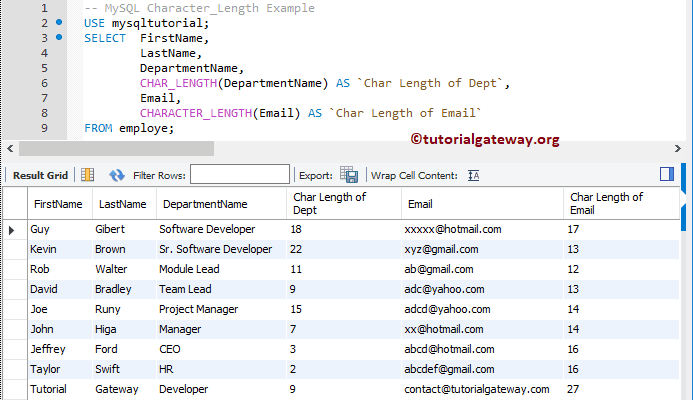# MySQL Char_Length Function

MySQL Char_Length function one of the String Function, which returns the length of the user-specified string measured in characters. This function considers the multi-byte character as a single character. And this is the significant difference between Char_Length and Length function. In MySQL, Character_length is the synonyms for the standard Character Length function. So, you can use any of these function names

## MySQL Char_Length Syntax

The basic syntax of Character_Length in MySQL is as shown below:

```SELECT LENGTH (String_Expression)
FROM Source```

To write this MySQL Character_Length Function using Command Prompt and Workbench, we are going to use the below shown data

## MySQL Char_Length Example 1

The char length function is to count the number of single characters inside a specified expression. The following query shows you multiple ways to use this character length function.

```-- MySQL Character Length Example
SELECT CHAR_LENGTH ('MySQL') AS `Char Length`;

SELECT CHAR_LENGTH ('Learn MySQL Tutorials') AS `MySQL Length`;

SELECT CHAR_LENGTH ('Tutorial Gateway') AS `Website Length`;```

Remember, the Char_Length function counts the empty space as well.

## MySQL Char_Length Example 2

The char_length function also allows you to find the character length of the data inside a column. In this MySQL example, we are going to find the character length of two columns: Department Name and Email column.

```-- MySQL Character_Length Example
USE mysqltutorial;
SELECT  FirstName,
LastName,
DepartmentName,
CHAR_LENGTH(DepartmentName) AS `Char Length of Dept`,
Email,
CHARACTER_LENGTH(Email) AS `Char Length of Email`
FROM employe;```## MySQL Char_Length Function WHERE Condition

In this String Function example, we show you how to use Character length function at where condition. Here, we used the MySQL char_length function in where clause to check whether the character Length of a department column data is greater than 7 or not.

```-- MySQL Character_Length Example
USE mysqltutorial;
SELECT  FirstName,
LastName,
DepartmentName,
CHAR_LENGTH(DepartmentName) AS `Char Length of Dept`,
Email,
CHARACTER_LENGTH(Email) AS `Char Length of Email`
FROM employe
WHERE CHAR_LENGTH(DepartmentName) > 7
ORDER BY `Char Length of Dept`;```

Remember, WHERE Clause does not understand the ALIAS Column name declared in the SELECT Statement. So, you have to use Character_Length function explicitly at where clause